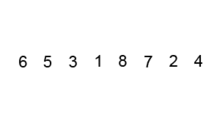# Bubble Sort, JavaScript

Hi there! Today, I want to start a series of articles about algorithms. Traditionally, we will begin laerning them with sorting one-dimensional arrays and even more traditionally – with bubble sort.

Bubble sort is also sometimes considered sinking sort.

Generally, the only advantage of this algorithm is that it is extremely simple to implement. Actually, it is not efficient, with O(n²) complexity and is not used in practice. However, it may become useful to know this kind of sort as other more complex and optimized algorithms are created based on it. For example, Cocktail sort, Heapsort, Quicksort, etc.

The algorithm essence is in comparing a pair of neighbouring elements – if they are in the wrong order, then they will be swapped. To sort the whole array of length N in this way, you will have to walk through it N-1 times (the last element will already be sorted at the previous iteration, so no pass-through is required).

Also, the largest number pops up to the end of the array for each iteration when ascending. Otherwise, in descending order — the smallest number pops up. This way, you do not need to to check it again at the next iteration.Bubble sort algorithm visualisation

In the first approximation, the algorithm for sorting in ascending order looks the following way:

function bubbleSort(arr) {

for (var i = 0, endI = arr.length – 1; i < endI; i++) {

for (var j = 0, endJ = endI – i; j < endJ; j++) {

if (arr[j] > arr[j + 1]) {

var swap = arr[j];

arr[j] = arr[j + 1];

arr[j + 1] = swap;

}

}

}

return arr;

}

However, in this example, it does not take into account the array entered. Even for an already sorted array, the application will need to execute all the loops’ iterations.

It can be optimized by adding a flag that tracks the elements’ scrambling. In case there is no scrambling for a certain pass-through – the array is already sorted. The code will now look as follows:

function bubbleSort(arr) {

for (var i = 0, endI = arr.length – 1; i < endI; i++) {

var wasSwap = false;

for (var j = 0, endJ = endI – i; j < endJ; j++) {

if (arr[j] > arr[j + 1]) {

var swap = arr[j];

arr[j] = arr[j + 1];

arr[j + 1] = swap;

wasSwap = true;

}

}

if (!wasSwap) break;

}

return arr;

}

Finally, let’s transfer our algorithm code to a new ES6 syntax and get rid of an additional variable:

const bubbleSort = arr => {

for (let i = 0, endI = arr.length – 1; i < endI; i++) {

let wasSwap = false;

for (let j = 0, endJ = endI – i; j < endJ; j++) {

if (arr[j] > arr[j + 1]) {

[arr[j], arr[j + 1]] = [arr[j + 1], arr[j]];

wasSwap = true;

}

}

if (!wasSwap) break;

}

return arr;

};

In case you have faced some difficulties with understanding the algorithm functioning, you can see its visualisation in the following videos:

<iframe width=”657″ height=”383″ src=”https://www.youtube.com/embed/lyZQPjUT5B4″ frameborder=”0″ allow=”accelerometer; autoplay; encrypted-media; gyroscope; picture-in-picture” allowfullscreen></iframe>

<iframe width=”657″ height=”383″ src=”https://www.youtube.com/embed/yIQuKSwPlro” frameborder=”0″ allow=”accelerometer; autoplay; encrypted-media; gyroscope; picture-in-picture” allowfullscreen></iframe>

We believe you have no questions currently:)

However, in case you have some – provide with feedback at comments.

Tags: